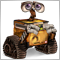# Problem with bool variable in MQL5130

I have a problem with a bool variable. I did the global declaration (outside the void OnTick() function) and then I need to use it later inside the void OnTick().

It's important because after checking conditions I will use the variable in the IF for checking the conditions to open a trade. For some reason always return false, and that shouldn't be the case.

```bool myvariable;

void OnTick()
{
//many lines of code

if (indicator1Array > indicator2Array && indicator3Array < indicator4Array)
myvariable = true;
else
myvariable = false;

if (indicator Array5 <  50 &&  myvariable==true && PositionsTotal()==0)
// Open Buy Order code
```

Is this logic correct? I can't understand what mistake I made here. Thank you!3176

patagonia2015:

I have a problem with a bool variable. I did the global declaration (outside the void OnTick() function) and then I need to use it later inside the void OnTick().

It's important because after checking conditions I will use the variable in the IF for checking the conditions to open a trade. For some reason always return false, and that shouldn't be the case.

Is this logic correct? I can't understand what mistake I made here. Thank you!

maybe it is never set to true ?130

Marco Montemari:

maybe it is never set to true ?

I check that, but rewriting the same code in a different manner (without the boolen variable and just have all the code in the IF that if true will generate a buy signal) works as intended, meaning it have trades.

Do you not see anything wrong with the code?

Thanks!18099

1. ```bool myvariable;

void OnTick(){```
It only needs to be global, if you reference it in multiple routines. Otherwise put it inside.
It only needs to be static, if you need to remember the value, between calls.

2. ```if (indicator1Array > indicator2Array && indicator3Array < indicator4Array)
myvariable = true;
else
myvariable = false;```
```bool isUpTrend = indicator1Array > indicator2Array
&& indicator3Array < indicator4Array;
```
Use meaningful variable names.

3. `if (indicator Array5 <  50 && isUpTrend==true && PositionsTotal()==0) `
You should be able to read your code out loud and have it make sense. You would never write if( (2+2 == 4) == true) would you? if(2+2 == 4) is sufficient. So don't write if(bool == true), just use if(bool) or if(!bool). Code becomes self documenting when you use meaningful variable names, like bool isLongEnabled where as Long_Entry sounds like a trigger price or a ticket number and "if long entry" is an incomplete sentence.

4. Print your variables and find out why.130

whroeder1:
1. It only needs to be global, if you reference it in multiple routines. Otherwise put it inside.
It only needs to be static, if you need to remember the value, between calls.

2. Simplify your codeUse meaningful variable names.

3. You should be able to read your code out loud and have it make sense. You would never write if( (2+2 == 4) == true) would you? if(2+2 == 4) is sufficient. So don't write if(bool == true), just use if(bool) or if(!bool). Code becomes self documenting when you use meaningful variable names, like bool isLongEnabled where as Long_Entry sounds like a trigger price or a ticket number and "if long entry" is an incomplete sentence.

4. Print your variables and find out why.

Thank you for your reply. I didn't know I could write Boolean variable conditions like that. How do you recommend to print the variable to see the value it have on each call of the EA?

Is there a way to see this information while I'm using a backtest? Or the only way is to add the expert to the chart and then wait to see the variable as "true"?